# Histogram notes in python with pandas and matplotlib

Here are some notes (for myself!) about how to format histograms in python using pandas and matplotlib. The defaults are no doubt ugly, but here are some pointers to simple changes to formatting to make them more presentation ready.

First, here are the libraries I am going to be using.

``````import pandas as pd
import numpy as np
import matplotlib
from matplotlib import pyplot as plt
from matplotlib.ticker import StrMethodFormatter
from matplotlib.ticker import FuncFormatter``````

Then I create some fake log-normal data and three groups of unequal size.

``````#generate three groups of differing size
n = 50000
group = pd.Series(np.random.choice(3, n, p=[0.6, 0.35, 0.05]))
#generate log-normal data
vals = pd.Series(np.random.lognormal(mean=(group+2)*2,sigma=1))
dat = pd.concat([group,vals], axis=1)
dat.columns = ['group','vals']``````

And note I change my default plot style as well. So if you are following along your plots may look slightly different than mine.

One trick I like is using `groupby` and describe to do a simple textual summary of groups. But I also like transposing that summary to make it a bit nicer to print out in long format. (I use spyder more frequently than notebooks, so it often cuts off the output.) I also show setting the pandas options to a print format with no decimals.

``````#Using describe per group
pd.set_option('display.float_format', '{:,.0f}'.format)
print( dat.groupby('group')['vals'].describe().T )``````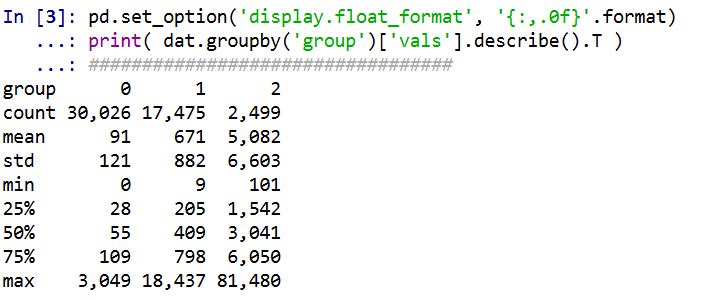Now onto histograms. Pandas has many convenience functions for plotting, and I typically do my histograms by simply upping the default number of bins.

``dat['vals'].hist(bins=100, alpha=0.8)``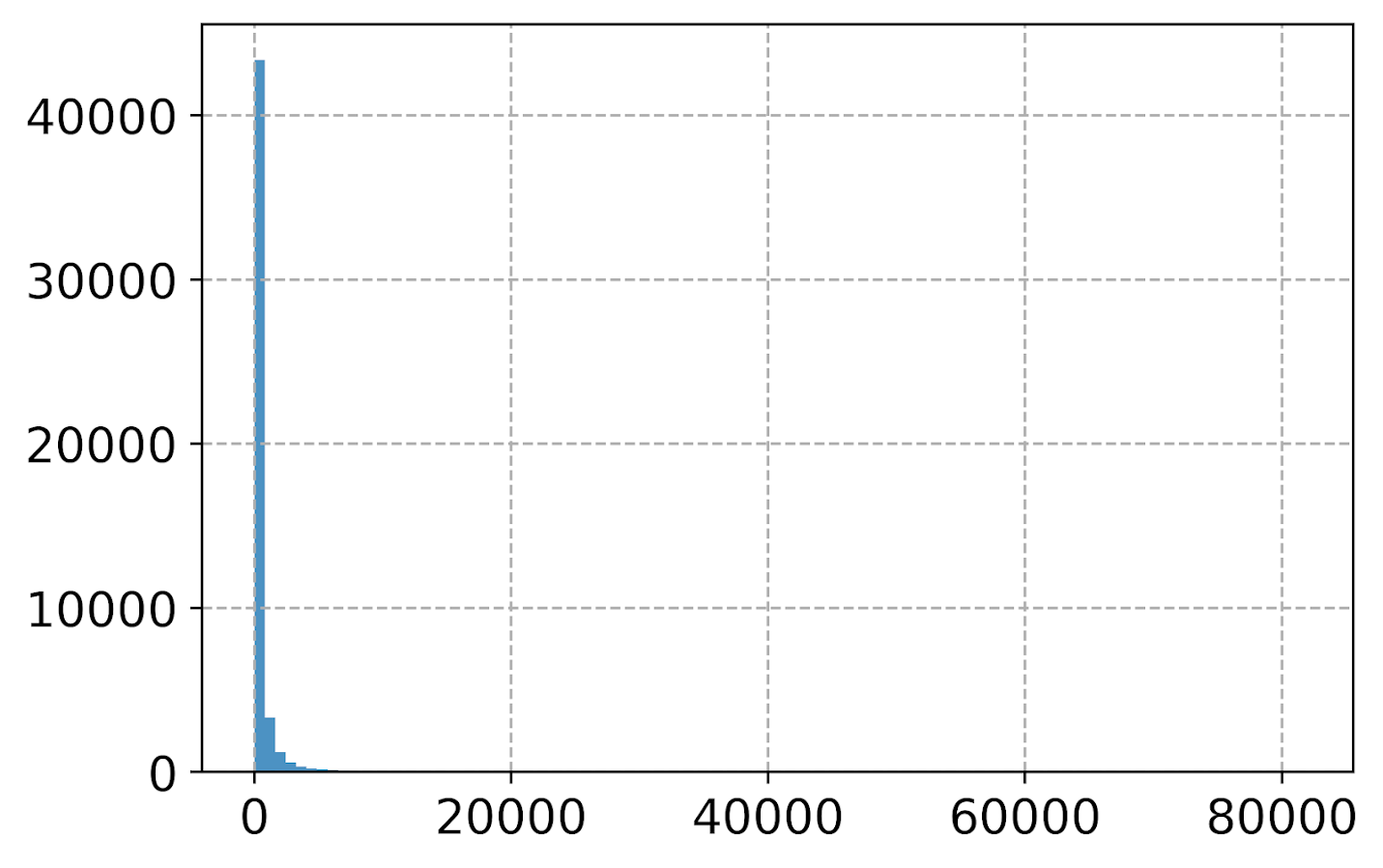Well that is not helpful! So typically when I see this I do a log transform. (Although note if you are working with low count data that can have zeroes, a square root transformation may make more sense. If you have only a handful of zeroes you may just want to do something like `np.log([dat['x'].clip(1))` just to make a plot on the log scale, or some other negative value to make those zeroes stand out.)

``````#Histogram On the log scale
dat['log_vals'] = np.log(dat['vals'])
dat['log_vals'].hist(bins=100, alpha=0.8)``````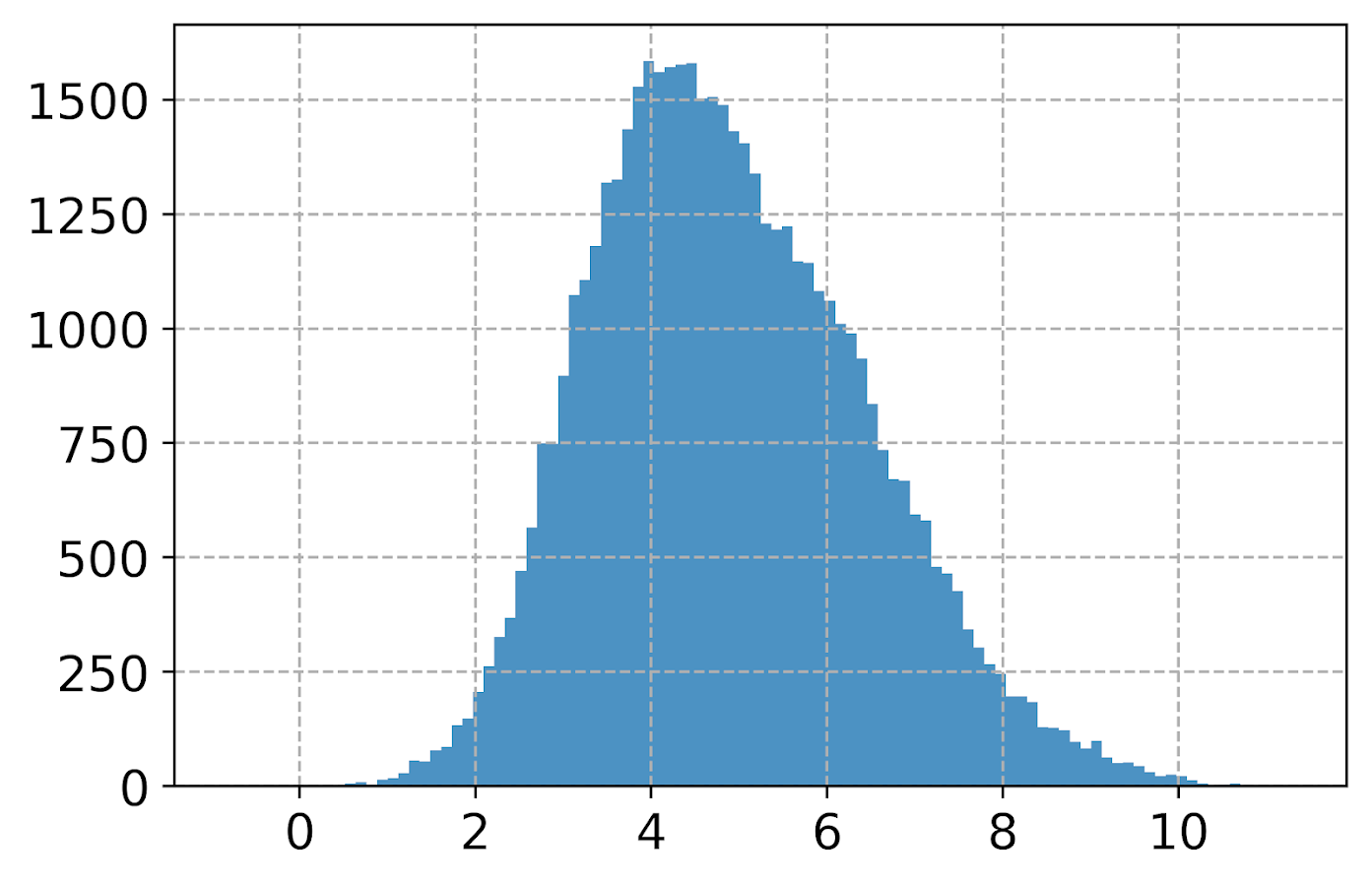Much better! It may not be obvious, but using pandas convenience plotting functions is very similar to just calling things like `ax.plot` or `plt.scatter` etc. So you can assign the plot to an axes object, and then do subsequent manipulations. (Don’t ask me when you should be putzing with axes objects vs plt objects, I’m just muddling my way through.)

So here is an example of adding in an X label and title.

``````#Can add in all the usual goodies
ax = dat['log_vals'].hist(bins=100, alpha=0.8)
plt.title('Histogram on Log Scale')
ax.set_xlabel('Logged Values')``````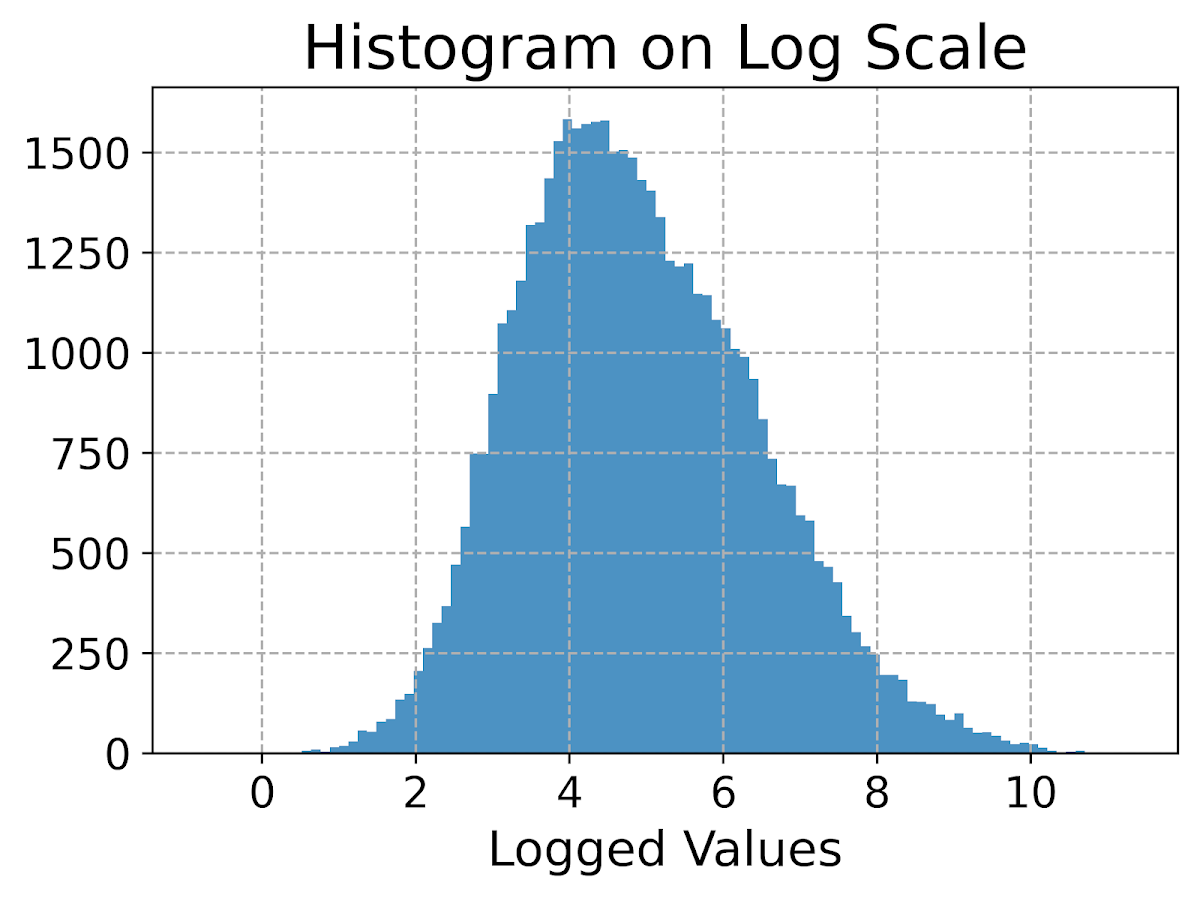Although it is hard to tell in this plot, the data are actually a mixture of three different log-normal distributions. One way to compare the distributions of different groups are by using `groupby` before the histogram call.

``````#Using groupby to superimpose histograms
dat.groupby('group')['log_vals'].hist(bins=100)``````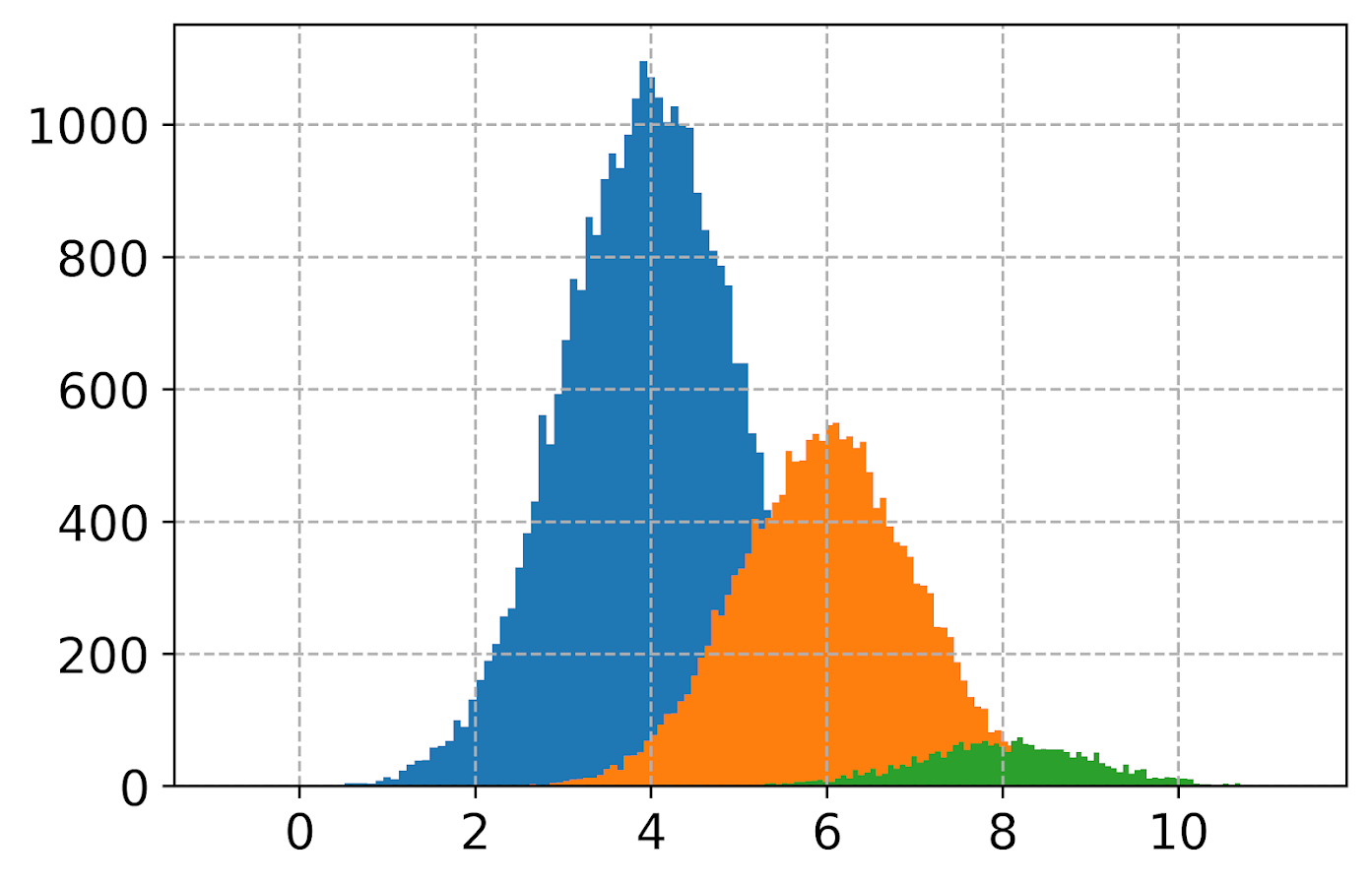But you see here two problems, since the groups are not near the same size, some are shrunk in the plot. The second is I don’t know which group is which. To normalize the areas for each subgroup, specifying the `density` option is one solution. Also plotting at a higher alpha level lets you see the overlaps a bit more clearly.

``dat.groupby('group')['log_vals'].hist(bins=100, alpha=0.65, density=True)``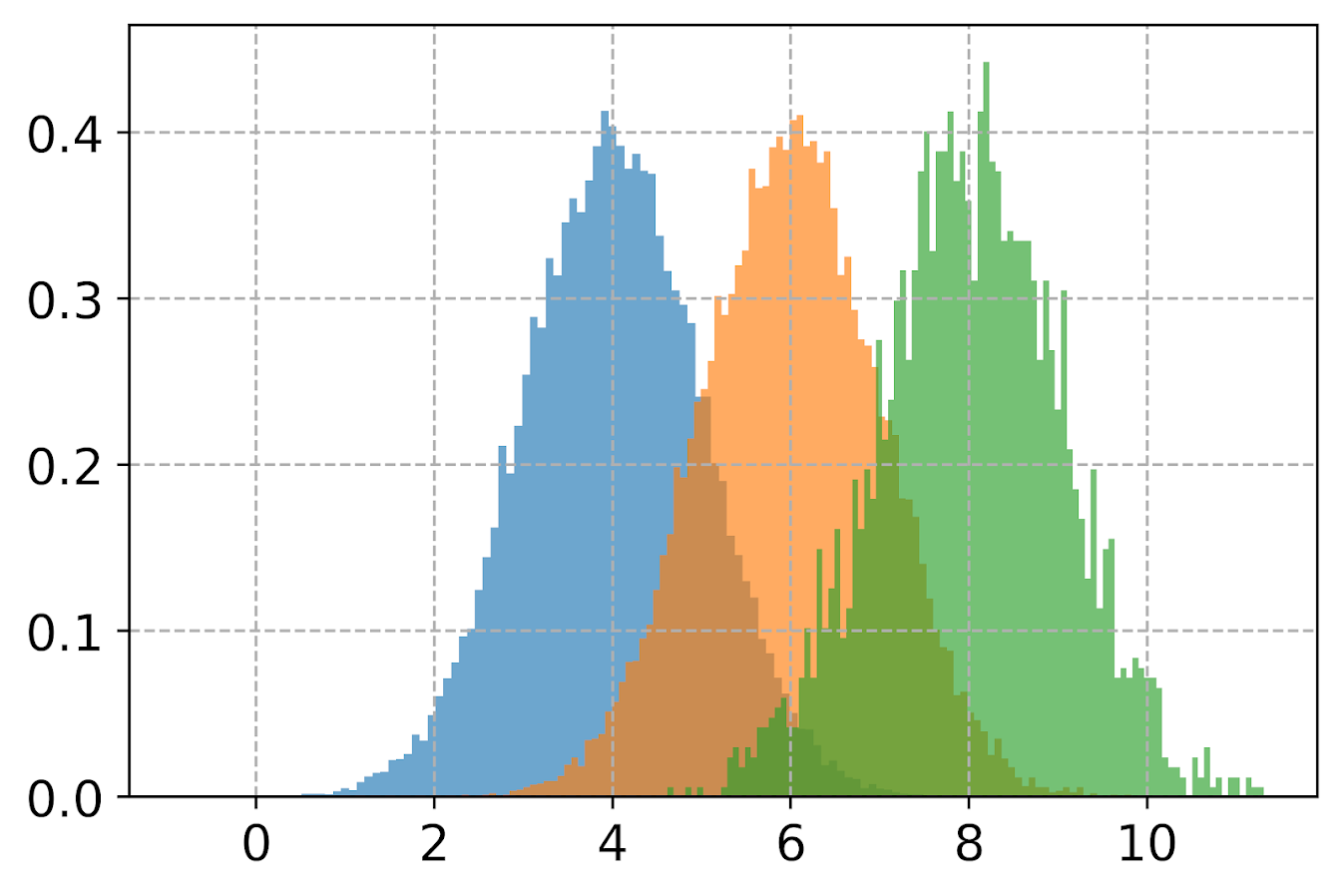Unfortunately I keep getting an error when I specify `legend=True` within the `hist()` function, and specifying `plt.legend` after the call just results in an empty legend. So another option is to do a small multiple plot, by specifying a `by` option within the `hist` function (instead of `groupby`).

``````#Small multiple plot
dat['log_vals'].hist(bins=100, by=dat['group'],
alpha=0.8, figsize=(8,8))``````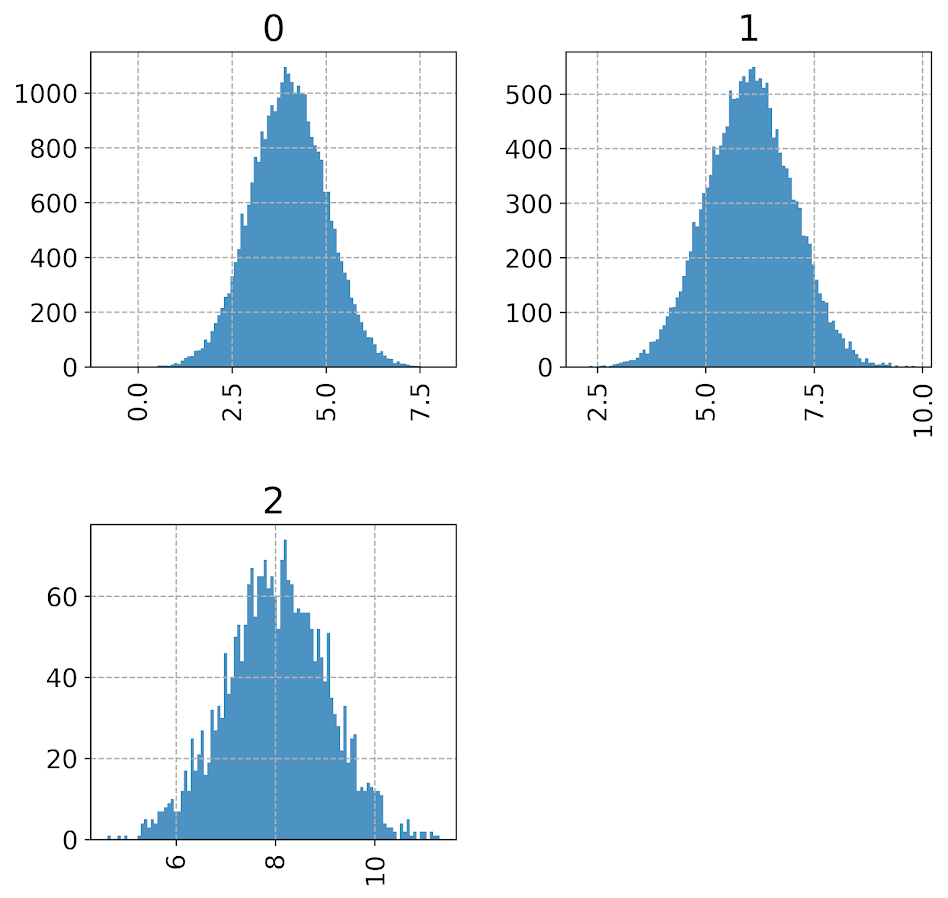This takes up more room, so can pass in the `figsize()` parameter directly to expand the area of the plot. Be careful when interpreting these, as all the axes are by default not shared, so both the Y and X axes are different, making it harder to compare offhand.

Going back to the superimposed histograms, to get the legend to work correctly this is the best solution I have come up with, just simply creating different charts in a loop based on the subset of data. (I think that is easier than building the legend yourself.)

``````#Getting the legend to work!
for g in pd.unique(dat['group']):
dat.loc[dat['group']==g,'log_vals'].hist(bins=100,alpha=0.65,
label=g,density=True)
plt.legend(loc='upper left')``````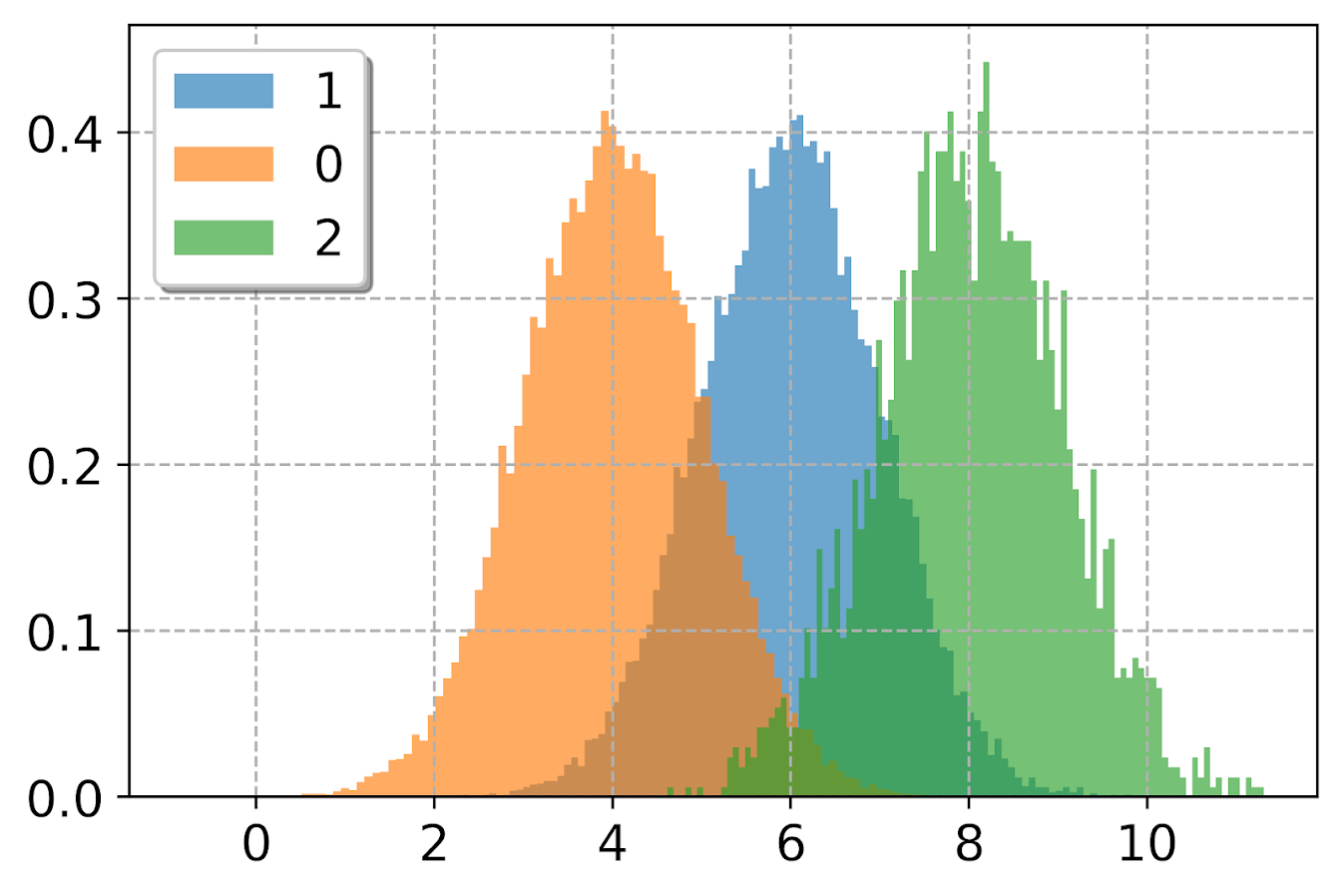Besides the `density=True` to get the areas to be the same size, another trick that can sometimes be helpful is to weight the statistics by the inverse of the group size. The Y axis is not really meaningful here, but this sometimes is useful for other chart stats as well.

``````#another trick, inverse weighting
dat['inv_weights'] = 1/dat.groupby('group')['vals'].transform('count')
for g in pd.unique(dat['group']):
sub_dat = dat[dat['group']==g]
sub_dat['log_vals'].hist(bins=100,alpha=0.65,
label=g,weights=sub_dat['inv_weights'])
plt.legend(loc='upper left')    ``````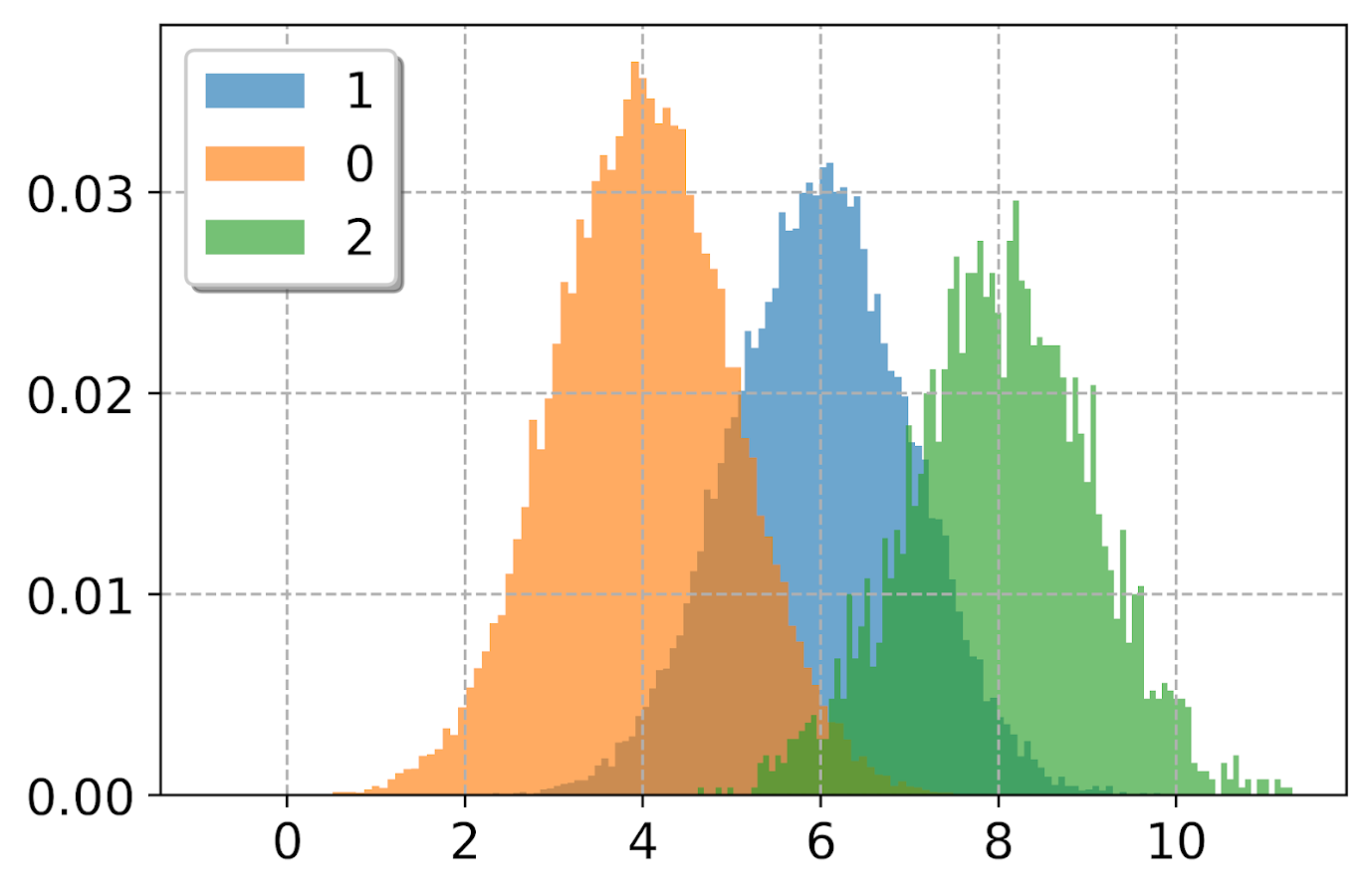So far, I have plotted the logged values. But I often want the labels to show the original values, not the logged ones. There are two different ways to deal with that. One is to plot the original values, but then use a log scale axis. When you do it this way, you want to specify your own bins for the histogram. Here I also show how you can use `StrMethodFormatter` to return a money value. Also rotate the labels so they do not collide.

``````#Specifying your own bins on original scale
#And using log formatting
log_bins = np.exp(np.arange(0,12.1,0.1))
ax = dat['vals'].hist(bins=log_bins, alpha=0.8)
plt.xscale('log', basex=10)
ax.xaxis.set_major_formatter(StrMethodFormatter('\${x:,.0f}'))
plt.xticks(rotation=45)``````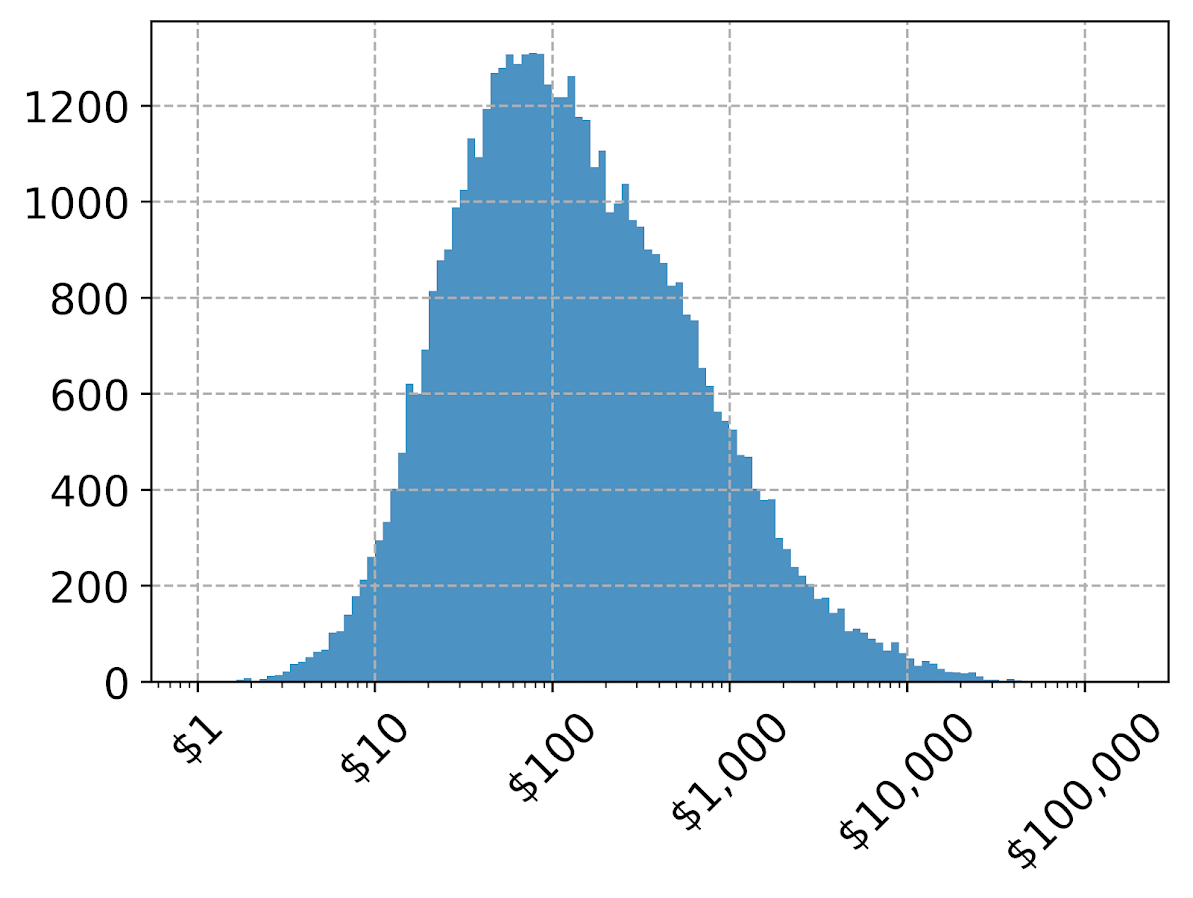If you omit the formatter option, you can see the returned values are `10^2`, `10^3` etc. Besides log base 10, folks should often give log base 2 or log base 5 a shot for your data.

Another way though is to use our original logged values, and change the format in the chart. Here we can do that using `FuncFormatter`.

``````#Using the logged scaled, then a formatter
#https://napsterinblue.github.io/notes/python/viz/tick_string_formatting/
def exp_fmt(x,pos):
return '\${:,.0f}'.format(np.exp(x))
fmtr = FuncFormatter(exp_fmt)

ax = dat['log_vals'].hist(bins=100, alpha=0.8)
plt.xticks(np.log([5**i for i in range(7)]))
ax.xaxis.set_major_formatter(fmtr)
plt.xticks(rotation=45)``````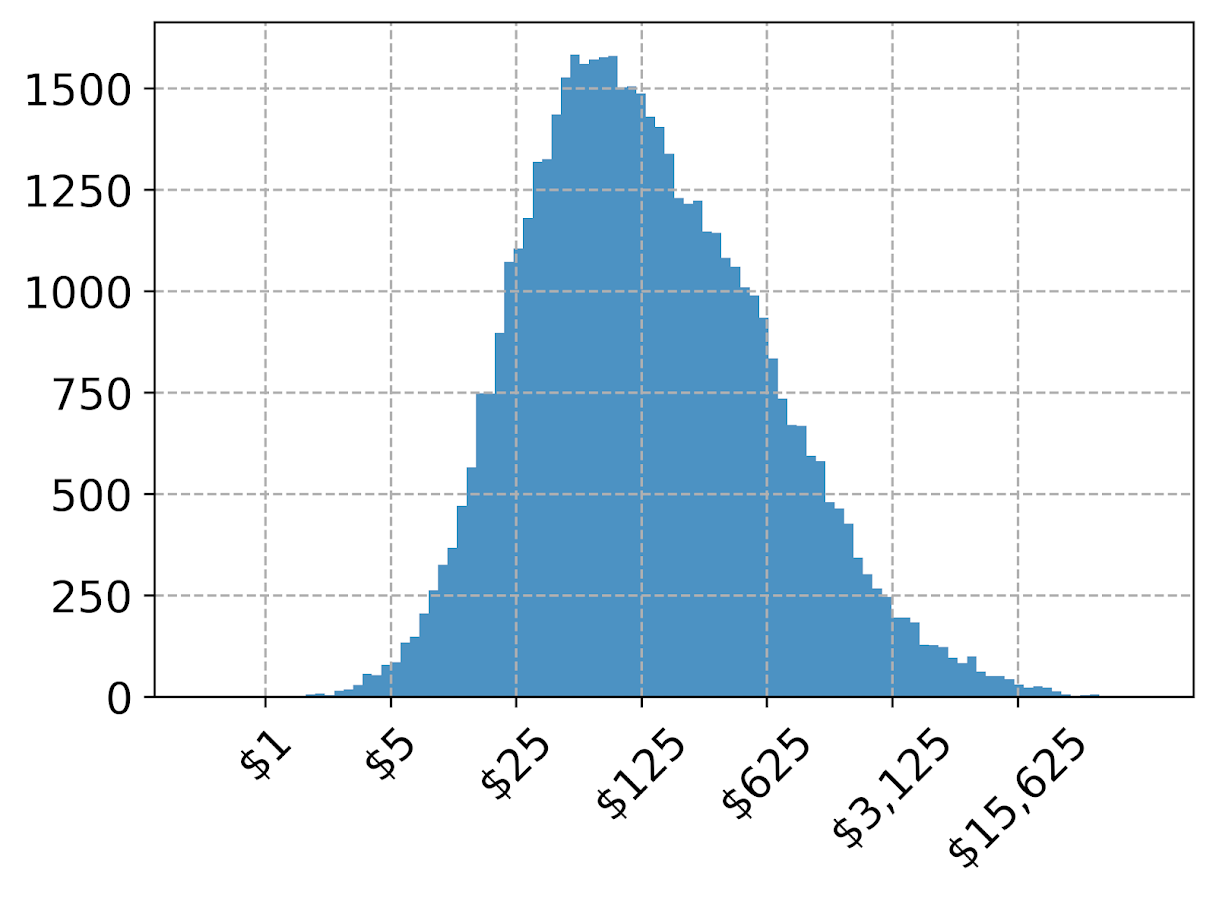On the slate is to do some other helpers for scatterplots and boxplots. The panda defaults are no doubt good for EDA, but need some TLC to make more presentation ready.

# The baseline in histograms & outliers

One of my current reads is Graphics of Large Datasets: Visualizing a Million (Unwin, Theus & Hofman, 2007). In one of the introductory chapters (I believe it was Theus) makes the point that for histograms with stretched out values it is very difficult to identify outliers in the tails (or really see the density at all).

Here is an example with the crime data for 21,506 street units I am using for my dissertation.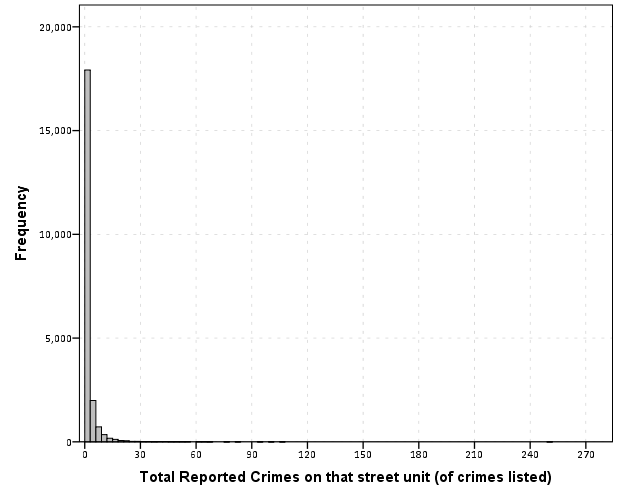Now we know there likely are a few outliers based on SPSS drawing the chart axis to 300, but we can not see their location. The bar lengths are so tiny that they are indiscriminable from the outline of the chart. In very large datasets, the height of the bar is not even guaranteed to encompass one pixel on the screen (depending on the Y axis scale).

But most statistical packages draw the bars with outlines, so even if the height of the bar won’t necessarily have any pixels devoted to it, most histograms will still spare some ink to draw the outline. So I immediately thought an simple improvement to this same chart would be instead of anchoring the bins to the bottom of the chart, simply add a bit of buffer below the baseline so there is some whitespace between the histogram bars and the chart outline.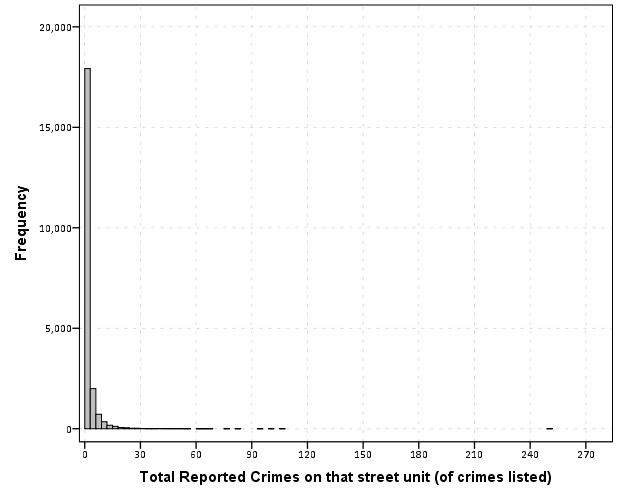So now we can see that we have a lone outlier around 250 crimes on the street and no others within 100 crimes. The density taking into account the outline of the bar may be inaccurate in an absolute sense, but it is really an inconsequential error in terms of evaluating the shape of the distribution.

Clearly histograms are not the most appropriate tool for identifying outliers (e.g. a rug plot showing individual values below the axis would help), but this is a fairly simple change to make the typical histogram more informative. In SPSS you can simply edit the chart interactively to give the Y axis a buffer below the lowest value. The same advice applies to bar charts as well with low values in certain categories. Knowing the difference between very few and 0 is an important distinction both for histograms and bar charts.

Maybe histograms and bar charts should be drawn with this whitespace buffer by default.